# QUADRATIC EQUATION - NATURE OF ROOTS

QUADRATIC EQUATION - NATURE OF ROOTS

a.

Consider the quadratice quation ax2 +bx+c=0 wherea,b,c  belongs to R & a != 0then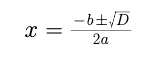(i)  D > 0 i.e roots are real & distinct (unequal).

(ii)  D = 0 i.e roots are real & coincident (equal)

(iii)  D < 0 i.e roots are imaginary.

(iv)  If p + i q is one root of a quadratic equation, then the other root must be the conjugate

p–i q & vice versa. (p,q belongs R &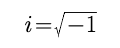).

b.

Consider the quadratice quation ax2 +bx+c=0 wherea,b,c  belongs to Q & a != 0 then

(i)  If D is a perfect square, then roots are rational.

(ii) If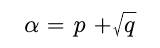is one root in this case,(where p is rational & q is a surd)  then other root will be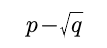Example :

If the coefficient of the quadratic equation are rational & the coefficient of x2 is 1, then find the equation one of whose roots is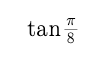Solution :

We know that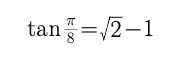Irrational roots always occur in conjugational pairs

Hence if one root is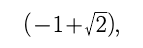the other root will be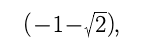equation is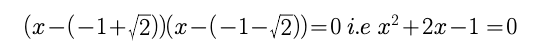Example :

Find all the integral values of a for which the quadratic equation (x – a)(x – 10) + 1 = 0 has integral roots.

Splution :

Here the equation is x2 – (a + 10)x + 10a + 1 = 0. Since integral roots will always be rational it means D should be a perfect square.

From(i)

D=a2 –20a+96.
i.e  D=(a–10)(a-10) –4  i.e 4=(a–10)(a-10) –D

If D is a perfect square it means we want difference of two perfect square as 4 which is possible only when

(a–10)(a-10) =4 and D=0.

i.e  (a–10) = ±2 so  a= 12, 8

Post By : Rahul Kumar 05 Apr, 2020 1838 views Maths